# Automatic Number Plate Recognition in Shogun¶

#### By Abhijeet Kislay (GitHub ID: kislayabhi)¶

This notebook is about performing ANPR to detect automobile license plates in photographs taken between 1-2 metres from a car. We will be introduced to techniques related to image segmentation, feature extraction, pattern recognition , and two important pattern recognition algorithms SVM and ANN.

## Background¶

Automatic Number Plate Recognition (ANPR), is a surveillance method that uses Optical Character Recognition (OCR) and other methods such as segmentations and detection to read vehicle registration plates.

The best results in an ANPR system can be obtained with an infrared (IR) camera, because the segmentation steps for detection and OCR segmentation are easy, clean, and minimize errors. Sadly, we do not use IR photographs here. That is we are going to try and get same results with regular photographs only!

Each country has different license plate sizes and specifications; it is useful to know these specifications in order to get the best results and reduce errors. The algorithms used here are intended to explain the basics of ANPR and the specifications for license plates from Croatia (Why? because I found it's license plate database fairly easily on the internet), but we can extend them to any country or specification.(check references for the link)

I have loaded few of those plates here.

In :
from IPython.display import Image

Image(filename='../../../data/ANPR/sample_plates.png')

Out: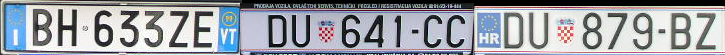The whole algorithmic approach is structured as following:

1. Plate Detection
2. Segmentation
3. Classification
4. Plate Recognition
5. OCR Segmentation
6. Feature Extraction
7. OCR Classification

We will go through each of these steps one by one.

## 1. Plate Detection¶

First load a car image. It's better to see what we are dealing with

In :
#Use the following function when reading an image through OpenCV and displaying through plt.
def showfig(image, ucmap):
#There is a difference in pixel ordering in OpenCV and Matplotlib.
#OpenCV follows BGR order, while matplotlib follows RGB order.
if len(image.shape)==3 :
b,g,r = cv2.split(image)       # get b,g,r
image = cv2.merge([r,g,b])     # switch it to rgb
imgplot=plt.imshow(image, ucmap)
imgplot.axes.get_xaxis().set_visible(False)
imgplot.axes.get_yaxis().set_visible(False)

#import Opencv library
try:
import cv2
except ImportError:
print "You must have OpenCV installed"
import matplotlib.pyplot as plt
import numpy as np
%matplotlib inline

plt.rcParams['figure.figsize'] = 10, 10

# Actual Code starts here
plt.title('Sample Car')
image_path="../../../data/ANPR/sample_1.jpg"
carsample=cv2.imread(image_path)
showfig(carsample,None)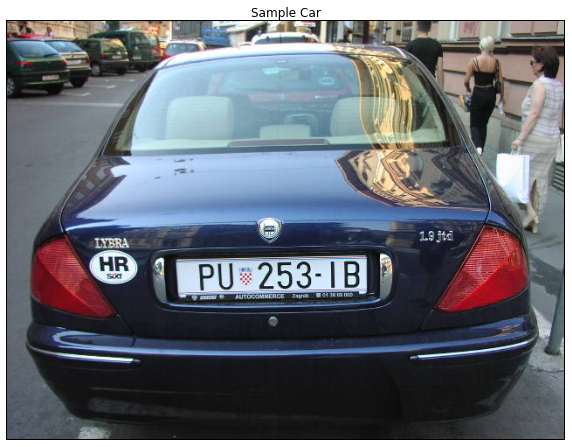In this step we have to detect all the plates in the current camera frame. Two broad categories in which they can be defined are:

1. Segmentation
2. Classification

### Segmentation¶

Segmentation is the process of dividing an image into multiple segments. This process is to simplify the image for analysis and make feature extraction easier.

One important feature that we can exploit from Number plates are the high number of vertical edges. But before that, we need to do some handy preprocessing of the current image namely:

1. grayscale conversion : color won't help us in this task
2. Remove Noise : A 5x5 Gaussian blur to remove unwanted vertical edges

To find the vertical edges, we will use a Sobel filter and find its first horizontal derivative.

In :
plt.rcParams['figure.figsize'] = 7,7

# convert into grayscale
gray_carsample=cv2.cvtColor(carsample, cv2.COLOR_BGR2GRAY)
showfig(gray_carsample, plt.get_cmap('gray'))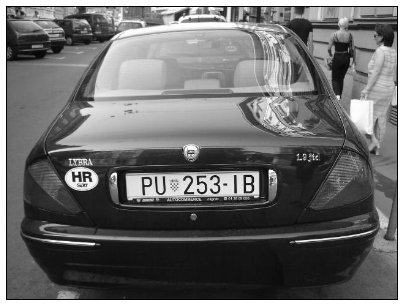In :
# blur the image
blur=cv2.GaussianBlur(gray_carsample,(5,5),0)
showfig(blur, plt.get_cmap('gray'))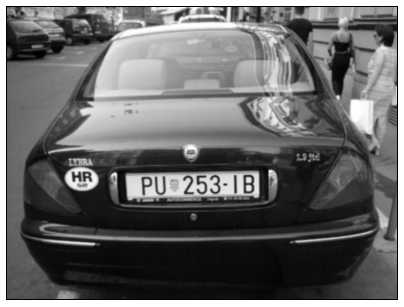In :
# find the sobel gradient. use the kernel size to be 3
sobelx=cv2.Sobel(blur, cv2.CV_8U, 1, 0, ksize=3)
showfig(sobelx, plt.get_cmap('gray'))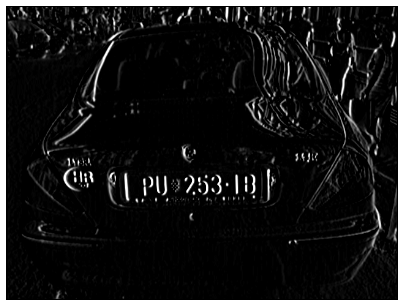After a Sobel Filter, we apply a threshold filter to obtain a binary image with a threshold value obtained through Otsu's Method.Otsu's algorithm needs an 8-bit input image and Otsu's method automatically determines the optimal threshold value:

In :
#Otsu thresholding
_,th2=cv2.threshold(sobelx, 0, 255, cv2.THRESH_BINARY+cv2.THRESH_OTSU)
showfig(th2, plt.get_cmap('gray'))